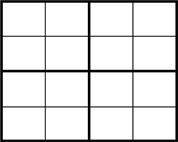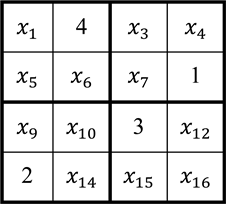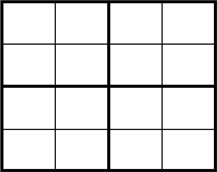﻿ 吴方法在求解数独问题中的应用

# 吴方法在求解数独问题中的应用The Application of Wu’s Method of Characteristic Set in Solving Sudoku Problem

Abstract: Wu’s method is a crucial method in computer algebra. It achieves the elimination purpose by cal-culating the Wu’s characteristic sets. The irreducible characteristic series is a set of triangular sets obtained by factoring the Wu’s characteristic sets, which can provide the zero decomposition of the polynomial equations. In this paper, the Sudoku is modeled by analogy with graph coloring problem, and a method to express Sudoku with polynomial equations is given. Taking Shidoku as an example, we compute the irreducible characteristic series of its polynomial equations and some conclusions are listed.

1. 引言

2. 数独问题的多项式表示Figure 1. Shidoku puzzle without clues

1) 如上图1设出16个变量 ${x}_{1},{x}_{2},\cdots ,{x}_{16}$，分别表示16个格位，用有限域 ${\mathbb{F}}_{11}$ 中的四个元素 $\left\{1,2,3,4\right\}$ 分别表示要填的四个数字(注：选择在有限域 ${\mathbb{F}}_{11}$ 中进行计算，是因为我们所应用的计算软件Singular分别用 $\left\{-\frac{p-1}{2},-1,0,1,\cdots ,\frac{p-1}{2}\right\}$ 这11个元素来表示 ${\mathbb{F}}_{p}$ (其中p为素数)中个元素， $p=11$ 是使 ${\mathbb{F}}_{p}$ 包含数字1，2，3，4的最小的素数了，为了读写方便，所以选择在 ${\mathbb{F}}_{11}$ 中进行计算。)；

2) 写出表示格位取值的16个多项式： $F\left({x}_{i}\right)={\prod }_{n=1}^{4}\left({x}_{i}-n\right),\text{\hspace{0.17em}}\left(i=1,2,\cdots ,16\right)$

3) 若格位 ${x}_{i}$ 和格位 ${x}_{j}$ 处不能填相同的数字，则用 $G\left({x}_{i},{x}_{j}\right):=\frac{F\left({x}_{i}\right)-F\left({x}_{j}\right)}{{x}_{i}-{x}_{j}}$ 来表示，这样的方程共有56个，分别用来表示每行、每列、每宫内的格位中所填数字不能相同。

3. 吴特征列与不可约特征序列

$\mathcal{K}$ 是一个数域，F是多项式环 $\mathcal{K}\left[{x}_{1},\cdots ,{x}_{n}\right]$ (默认变元序为 ${x}_{1}<\cdots <{x}_{n}$ )中的一个多项式。我们定义，多项式F中所含的变元的最大下标 $cls\left(F\right):=\text{max}\left\{k:\mathrm{deg}\left(F,{x}_{k}\right)>0,1\le k\le n\right\}$ 为F的类，如果 $F\in \mathcal{K}$ 则规定 $cls\left(F\right)=0$。当 $cls\left(F\right)>0$ 时，把 $lv\left(F\right):={x}_{cls\left(F\right)}$ 称为是F的导元；把 $ldeg\left(F\right):=deg\left(F,lv\left(F\right)\right)$ 称为F的导次数；把 $ini\left(F\right):=lc\left(F,lv\left(F\right)\right)$ 称为F的初式。

$F,G$ 是多项式环 $\mathcal{R}\left[x\right]$ 中的任意两个多项式， ${x}_{k}$ 是一个变元，而且有 $l=deg\left(G,{x}_{k}\right)$$m=deg\left(F,{x}_{k}\right)$。如果 $l>0$，那么就存在多项式 $Q,R\in \mathcal{R}\left[x\right]$ 和整数 $0\le s\le m-l+1$ 使得 $lc{\left(G,{x}_{k}\right)}^{s}\cdot F=Q\cdot G+R$，而且有 $deg\left(R,{x}_{k}\right)。如果s固定，那么 $Q,R$ 是唯一确定的。证明参见  。

$P,Q\in \mathcal{K}\left[x\right]$ 是非零多项式。并且有 $Q\notin \mathcal{K}$。如果 $deg\left(P,lv\left(Q\right)\right)。那么就称P对Q是约化的。设多项式集合 $\mathcal{T}\subseteq \mathcal{K}\left[x\right]$ 是一个三角列， $P\in \mathcal{K}\left[x\right]$ 是任意多项式，如果P对 $\mathcal{T}$ 中的每一个多项式T都是约化的，那么称P对 $\mathcal{T}$ 是约化的。

$\mathcal{P}\subseteq \mathcal{K}\left[x\right]$ 是非空多项式集合，任意包含于由 $\mathcal{P}$ 所生成的理想的升列都称为 $\mathcal{P}$ 的中间列。

$\mathcal{P}\subseteq \mathcal{K}\left[x\right]$ 是非空多项式集合，如果存在升列 $\mathcal{C}$ 使得 $\mathcal{C}\subseteq 〈\mathcal{P}〉$ 而且 $prem\left(\mathcal{P},\mathcal{C}\right)=\left\{0\right\}$，那么就称 $\mathcal{C}$$\mathcal{P}$ 的特征列。

$P,Q\in \mathcal{K}\left[x\right]$ 是非零多项式，如果满足下列三个条件之一，则称P的秩低于Q的秩，记为 $P\prec Q$ ：1) $P\in \mathcal{K}$，但是 $Q\notin \mathcal{K}$；2) $P,Q\notin \mathcal{K}$，而且 $cls\left(P\right)；3) $P,Q\notin \mathcal{K}$$cls\left(P\right)=cls\left(Q\right)$，而且 $ldeg\left(P\right)。如果 $Q\prec P$，则称P的秩高于Q的秩，记为 $P\succ Q$。如果 $P\prec Q$$P\succ Q$ 都不成立，那么称P和Q具有相同的秩，记为 $P~Q$。把 $P\prec Q$$P~Q$ 简记为 $P\precsim Q$

$\mathcal{T}=\left[{T}_{1},\cdots ,{T}_{r}\right]$$\mathcal{S}=\left[{S}_{1},\cdots ,{S}_{t}\right]$ 是多项式环 $\mathcal{K}\left[x\right]$ 中的两个非平凡升列，称 $\mathcal{T}$ 的秩低于 $\mathcal{S}$ 的秩，记为 $\mathcal{T}\prec \mathcal{S}$，如果满足下列条件之一：1) 存在 $i\le \text{min}\left(r,t\right)$，使得对于每个 $j 都有 ${T}_{j}~{S}_{j}$，但是 ${T}_{i}\prec {S}_{i}$

2) $r>t$，而且对于每个 $j\le t$ 都有 ${T}_{j}~{S}_{j}$ 成立。如果 $\mathcal{S}\prec \mathcal{T}$，那么称 $\mathcal{T}$ 的秩高于 $\mathcal{S}$ 的秩，记为 $\mathcal{T}\succ \mathcal{S}$。如果 $\mathcal{T}\succ \mathcal{S}$$\mathcal{T}\prec \mathcal{S}$ 都不成立，那么称 $\mathcal{T}$$\mathcal{S}$ 具有相同的秩，记为 $\mathcal{T}~\mathcal{S}$。将 $\mathcal{T}\prec \mathcal{S}$ 或者 $\mathcal{T}~\mathcal{S}$ 简记为 $\mathcal{T}\precsim \mathcal{S}$。并规定平凡升列的秩总是低于非平凡升列的秩。

$\mathcal{T}=\left[{T}_{1},\cdots ,{T}_{r}\right]$$\mathcal{S}=\left[{S}_{1},\cdots ,{S}_{t}\right]$ 是多项式环 $\mathcal{K}\left[x\right]$ 中的两个升列，那么我们有下列结论成立：(1) $\mathcal{T}~\mathcal{S}$ 当且仅当 $r=t$ 而且对于所有 $j\le t$，都有 ${T}_{j}~{S}_{j}$ 成立；(2)设 $P\in \mathcal{K}\left[x\right]\\mathcal{K}$，并记 $v=lv\left(P\right)$，如果 $\mathcal{T}$ 是非平凡升列，并且P对于 $\mathcal{T}$ 是约化的，那么 $\left({\mathcal{T}}_{

$\mathcal{P}$ 的基列可以通过如下步骤找出：设 ${\mathcal{F}}_{1}:=\mathcal{P}$，令 ${B}_{1}$${\mathcal{F}}_{1}$ 中秩最低的多项式。如果 ${B}_{1}$ 是非零常数，那么 $\left[{B}_{1}\right]$ 就是 $\mathcal{P}$ 的一个基列。否则，令 ${\mathcal{F}}_{2}:=\left\{F\in {\mathcal{F}}_{1}\{B}_{1}:F对{B}_{1}是约化的\right\}$。如果 ${\mathcal{F}}_{2}=\varnothing$，那么 $\left[{B}_{1}\right]$ 就是

$\mathcal{P}$ 的一个基列。否则，设 ${B}_{2}$${\mathcal{F}}_{2}$ 中秩最低的多项式。易知 ${B}_{1}\prec {B}_{2}$。可以类似地依次构造 ${\mathcal{F}}_{3},{\mathcal{F}}_{4},\cdots$，因为 $\mathcal{P}$ 是非空有限的多项式集合，而基列或是一个非零常数，或是作为一个三角列最多含有n个多项式，所以上述构造过程必定会在有限步之后终止，最后我们就可以得到 $\mathcal{P}$ 的一个基列。

$\mathcal{P}$ 的吴特征列的算法如下：1) 设 $\mathcal{F}:=\mathcal{P}$$\mathcal{R}:=\mathcal{P}$；2) 如果集合 $\mathcal{R}$ 非空，则求取 $\mathcal{R}$ 的一个基列 $\mathcal{C}$；3) 若 $\mathcal{C}$ 中含有 $\mathcal{K}$ 中的元素，则另 $\mathcal{R}:=\varnothing$，否则另 $\mathcal{R}:=\left\{prem\left(F,\mathcal{C}\right):F\in \mathcal{F}\\mathcal{C}\right\}\\left\{0\right\}$；4) 另 $\mathcal{F}:=\mathcal{P}\cup \mathcal{C}\cup \mathcal{R}$，并返回第2)步，直到集合 $\mathcal{R}$ 为空集为止。最后一次求出的基列 $\mathcal{C}$ 即为多项式组 $\mathcal{P}$ 的吴特征列。该算法的终止性及正确性可参见  。

$\Psi$$\mathcal{K}\left[x\right]$ 中由升列 ${\mathcal{C}}_{1},\cdots ,{\mathcal{C}}_{e}$ 构成的有限集合， $\mathcal{P}$$\mathcal{K}\left[x\right]$ 中的多项式集合，如果有零点分解 $Z\left(\mathcal{P}\right)={\cup }_{i=1}^{e}Z\left({\mathcal{C}}_{i}/{\mathcal{I}}_{i}\right)$ 成立，且 $prem\left(\mathcal{P},{\mathcal{C}}_{i}\right)=\left\{0\right\}$ 对于所有的i成立，则称 $\Psi$$\mathcal{P}$ 的特征序列。 $\Psi =\varnothing$$e=0$ 也即 $Z\left(\mathcal{P}\right)=\varnothing$

$\mathcal{T}\subseteq \mathcal{K}\left[x\right]$ 是一个三角列，则 $\mathcal{T}$ 可以写成如下形式：

$\mathcal{T}=\left[{T}_{1}\left({x}_{1},\cdots ,{x}_{{p}_{1}}\right),{T}_{2}\left({x}_{1},\cdots ,{x}_{{p}_{1}},\cdots ,{x}_{{p}_{2}}\right),\cdots ,{T}_{r}\left({x}_{1},\cdots ,{x}_{{p}_{1}},\cdots ,{x}_{{p}_{2}},\cdots ,{x}_{{p}_{r}}\right)\right]$ ,

$\mathcal{T}\subseteq \mathcal{K}\left[x\right]$ 是良好三角列，若 $\mathcal{T}$ 只含一个多项式 ${T}_{1}\left(u,{y}_{1}\right)$，且 ${T}_{1}$${\mathcal{K}}_{0}\left[{y}_{1}\right]$ 中的不可约多项式，则称 $\mathcal{T}$ 是不可约的。此时，设 ${\eta }_{1}$${T}_{1}$${\mathcal{K}}_{0}$ 的某一代数扩域中的零点，则称 $\left(u,{\eta }_{1}\right)$$\mathcal{T}$ 的一个一般零点。假设含任意 $k\left(k 个多项式的良好三角列之不可约性和一般零点已经定义，那么称良好三角列 $\mathcal{T}=\left[{T}_{1}\left(u,{y}_{1}\right),{T}_{2}\left(u,{y}_{1},{y}_{2}\right),\cdots ,{T}_{r}\left(u,{y}_{1},{y}_{2},\cdots ,{y}_{r}\right)\right]$ 是不可约的，如果 $\left[{T}_{1}\left(u,{y}_{1}\right),{T}_{2}\left(u,{y}_{1},{y}_{2}\right),\cdots ,{T}_{r-1}\left(u,{y}_{1},{y}_{2},\cdots ,{y}_{r-1}\right)\right]$ 是不可约的，且以 $\left(u,{\eta }_{1},\cdots ,{\eta }_{r-1}\right)$ 为一般零点，且多项式 ${T}_{r}\left(u,{y}_{1},{y}_{2},\cdots ,{y}_{r}\right)$${\mathcal{K}}_{r-1}\left[{y}_{r}\right]$ 中不可约。其中 ${\mathcal{K}}_{r-1}={\mathcal{K}}_{0}\left({\eta }_{1},\cdots ,{\eta }_{r-1}\right)$ 是将 ${\eta }_{1},\cdots ,{\eta }_{r-1}$ 添入 ${\mathcal{K}}_{0}$ 得到的代数扩域。此时设 ${\eta }_{r}$${T}_{r}\left(u,{y}_{1},{y}_{2},\cdots ,{y}_{r}\right)$${\mathcal{K}}_{r-1}$ 的某个代数扩域中的零点，称 $\left(u,{\eta }_{1},\cdots ,{\eta }_{r}\right)$$\mathcal{T}$ 的一个一般零点。

4. 应用及结论Figure 2. Shidoku puzzleFigure 3. Shidoku puzzle without clues

 Adams, W.W. and Loustaunau, P. (1994) An Introduction to Gröbner Bases. Graduate Studies in Mathematics, 3, 289 p.
https://doi.org/10.1090/gsm/003

 王东明. 消去法及其应用[M]. 北京: 科学出版社, 2002: 30-43, 111-125.

 王东明, 牟晨琪, 李晓亮, 等. 多项式代数[M]. 北京: 高等教育出版社, 2011: 65-88.

 Greuel, G.M. and Pfister, G. (2008) A Singular Introduction to Commutative Algebra. A Singular Introduction to Commutative Algebra. Springer, 571-646.

 Arnold, E., Lucas, S. and Taalman, L. (2010) Grobner Basis Representations of Sudoku. College Mathematics Journal, 41, 101-112.
https://doi.org/10.4169/074683410x480203

 Gago-Vargas, J., Hartillo-Hermoso, M.I. and Ucha-Enríquez, J.M. (2006) Sudokus and Gröbner Bases: Not Only a Divertimento. In: Ganzha, V.G., Mayr, E.W. and Vorozhtsov, E.V., Eds., Computer Algebra in Scientific Computing. CASC 2006. Lecture Notes in Computer Science, Vol. 4194, Springer, Berlin, Heidelberg, 155-165.
https://doi.org/10.1007/11870814_13

Top